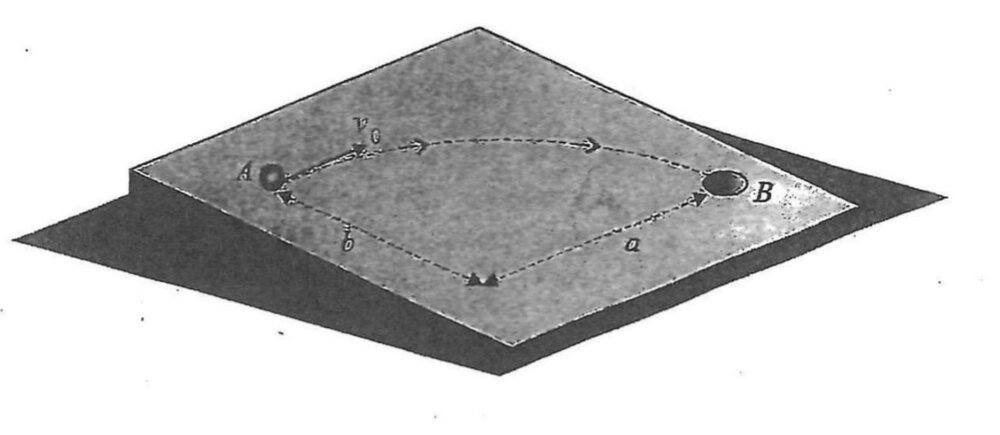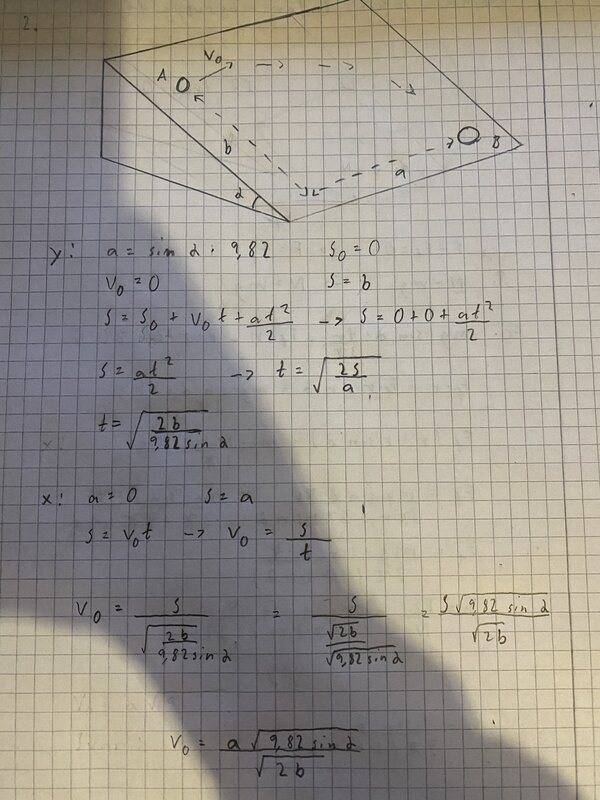# What velocity does the particle need to get to point B?

ClearWhey
Homework Statement:
A particle is at point A in a certain moment. The particle rolls with an angle of inclination a. It’s velocity direction is parallel to the upper edge. What velocity V0 does it need to reach into the hole B?

Sorry for my english
Relevant Equations:
Look at the picture to get a better idea of the problem.
It was a long time ago I did these kind of problems so I’m a bit rusty. The only thing I can think of is divide it up to two parts one x and one y.

In y the acceleration is sin(a)*9.82? Then put that in the equation and solve for t.

In x the there is no acceleration so the formula is x=V0*t, I put in t from y and solve for V0?

Haven’t gotten the answer paper yet so I have no idea if I’m on the right path or wrong. That is why I’m asking here if I’m solving this question right, if not how should I do it?

Thanks in advance for the help!Homework Helper
Gold Member
2022 Award
Looks like the right approach.

•ClearWhey
Mentor
You have given the inclination angle as ##a## and according to the figure the "horizontal" distance between A and B also labelled as ##a##. This might lead to confusing algebra.

Perhaps you meant to use ##\alpha## as the angle?

•ClearWhey
ClearWhey
You have given the inclination angle as ##a## and according to the figure the "horizontal" distance between A and B also labelled as ##a##. This might lead to confusing algebra.

Perhaps you meant to use ##\alpha## as the angle?

Yes you are correct.

Looks like the right approach.

What do you think about my solution?Homework Helper
Gold Member
2022 Award
What do you think about my solution?
That is right, but there is no point in substituting a numerical value for g when you don't have numbers for the rest of the variables. Just leave it as g.
And if you do substitute a numerical value you must include the units: ##v_0=a\sqrt{\frac{9.82\sin(\alpha)ms^{-2}}{2b}}##

•ClearWhey and etotheipi
ClearWhey
That is right, but there is no point in substituting a numerical value for g when you don't have numbers for the rest of the variables. Just leave it as g.
And if you do substitute a numerical value you must include the units: ##v_0=a\sqrt{\frac{9.82\sin(\alpha)ms^{-2}}{2b}}##

Alright will keep that in mind for the future and thanks for the help!

•jim mcnamara